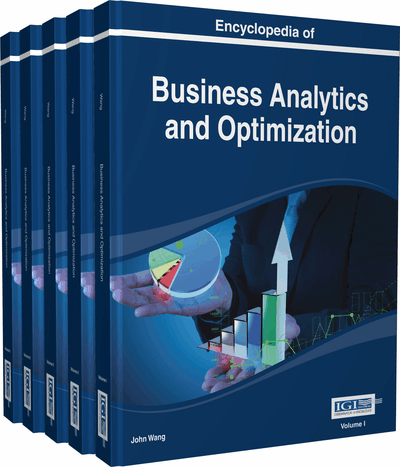# Simulation Optimization via Metamodeling Approach

Mehdi Zakerifar (University of Louisville, Louisville, USA), William E. Biles (University of Louisville, USA), Banu Y. Ekren (Izmir University of Economics, Turkey), Gerald W. Evans (University of Louisville, USA) and Sunderesh S. Heragu (Oklahoma State University, USA)
DOI: 10.4018/978-1-4666-5202-6.ch196

Top

## Introduction

Simulation optimization (SO) is the process of finding the best set of input variable values without explicitly evaluating each feasible set of these input variable values given an output criterion (Law, 2007; Fu, 1994). Input variables are called (controllable) inputs, parameter settings, values, variables, (proposed) solutions, designs, configurations, or factors (in design of experiments terminology). Outputs are called performance measures, criteria, or responses (in design of experiments terminology). Some of the outputs are used to form an objective function, and there is a constraint set on the inputs. Our aim is to optimize the objective function given below:

where f (θ) is the output criterion evaluated by simulation. θ is a controllable vector of p parameters. is a constraint set on θ.

SO has attracted many researchers. Because most real systems have numerous input variables and complex structure, evaluating each possible value of the input variables of such systems may become impossible. SO finds the best set of input variable values without explicitly evaluating each possible set of values.

In this chapter, we investigate SO techniques for infinite parameter space by focusing on metamodel-based approaches (see Figure 1). Here, we investigate discrete-event systems optimization for both continuous and discrete input variables (Nelson, 2010). In the following sections, we discuss two metamodeling approaches for the infinite parameter optimization procedure: Response Surface Methodology (RSM) and the Kriging metamodeling (KM).

Top

## Background

SO evaluates the outputs in the form of a “what if” question. Recently, computer technology advancement enabled us to answer “How to” questions as well, seeking optimum values for the decision variables so that a given output variable is maximized or minimized. There are several studies written on SO.

A comprehensive review of SO is provided by Fu (1994a, b). Later, Carson and Maria (1997) present a general summary of SO. Azadivar, Shu, and Ahmad (1996) implemented an SO algorithm on Box’s complex search method to optimize the locations and inventory levels in a production system. Pierreval and Tautou (1997) propose a new SO approach using evolutionary algorithms for manufacturing systems. Azadivar (1999) proposes a survey of issues on SO. Olafsson and Kim (2001) provide many techniques for considering discrete decision variables that have been suggested in solving SO problems. April, Glover, Kelly, and Laguna (2003) summarize some of the most relevant SO approaches and present an implementation for a real world project selection problem. Angelis, Felici, & Impelluso (2003) propose a methodology interactively using simulation, prediction of objective function and optimization to calculate and validate an optimal configuration of servers of a healthcare system.

Piera, Guasch, and Riera (2004) propose a petri net-based approach for SO which also uses information from the simulation model. Jung, Blau, Pekny, Reklaitis, and Eversdyk (2004) determine safety stock levels using deterministic planning and scheduling models to optimize customer satisfaction via an SO approach. Fu, Glover, and April (2005) provide a comprehensive review for SO. Fu, Chen, & Shi (2008) also present a tutorial introduction for SO. In that study, they classify the problem according to the decision variables and constraints, place the settings in the simulation context and summarize the approaches of SO.

## Key Terms in this Chapter

Input Variables: Also called (controllable) inputs, parameter settings, values, variables, (proposed) solutions, designs, configurations, or factors (in design of experiments terminology).

Infinite Parameter Space: Input variable values can get infinite number of values.

Metamodel: It is estimating of an input/output function that is defined by an underlying simulation model.

Response Surface Methodology: It is a technique to optimize the obtained metamodel using simulation.

Krigging: It is an interpolation method that predicts unknown values of a random function.

Outputs: Also called performance measures, criteria, or responses (in design of experiments terminology).

Simulation Optimization: It is the optimization of an output by finding the best input variable values from among all possibilities without explicitly evaluating each possibility of these input variable values.

Discrete Event Simulation: It is the simulation of a system where events occur at an instant in time changing the state of the system.

## Complete Chapter List

Search this Book:
Reset